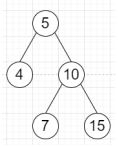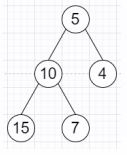ADBlock is blocking some content on the site# Program to invert a binary tree in Python

PythonServer Side ProgrammingProgramming

Suppose we have a binary tree root, we have to invert it so that its left subtree and right subtree are exchanged and their children are also exchanged recursively.

So, if the input is likethen the output will beTo solve this, we will follow these steps −

• Define a method solve(), this will take node

• if root is null, then

• return

• left of root := solve(right of root)

• right of root := solve(right of root)

• return root

Let us see the following implementation to get better understanding −

## Example

```class TreeNode:
def __init__(self, value):
self.val = value
self.left = None
self.right = None

def inorder(root):
if root:
inorder(root.left)
print(root.val, end=', ')
inorder(root.right)

class Solution:
def solve(self, root):
if not root:
return
root.left, root.right = self.solve(root.right), self.solve(root.left)
return root

ob = Solution()
root = TreeNode(5)
root.left = TreeNode(4)
root.right = TreeNode(10)
root.right.left = TreeNode(7)
root.right.right = TreeNode(15)
inv = ob.solve(root)
inorder(inv)```

## Input

```root = TreeNode(5)
root.left = TreeNode(4)
root.right = TreeNode(10)
root.right.left = TreeNode(7)
root.right.right = TreeNode(15)```

## Output

`15, 10, 7, 5, 4,`